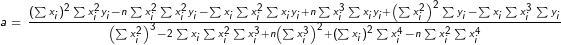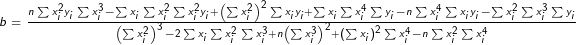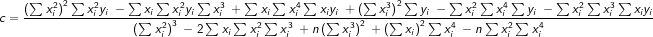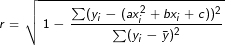This Quadratic Regression Calculator quickly and simply calculates the equation of the quadratic regression function and the associated correlation coefficient. In addition, it generates a scatter plot that depicts the curve of best fit.

You can use the quadratic regression calculator in three simple steps:

1. Input all known X and Y variables in the respective fields.
2. Click on the "Calculate" button to compute the quadratic regression equation.
3. Click on the "Reset" button to clear all fields and input new values.

### The Quadratic Regression Calculator uses the following formulas:

Quadratic regression: y = ax2 + bx + c, where a ≠ 0

Coefficients (a, b, c):Mean x: x̄ = ∑xi / n

Mean y: ȳ = ∑yi / n

Correlation coefficient r:Where:

n  is the total number of samples,

xi (x1, x2, ... ,xn) are the x values,

yi (y1, y2, ... ,yn) are the y values,

∑xi  is the sum of x values,

∑yi  is the sum of y values,

∑xi yi  is the sum of products of x and y values,

∑xi2  is the sum of squares of x values,

∑xi3  is the sum of the cubes of x values,

∑xi4  is the sum of the fourth powers of x values.

You may also be interested in our Linear Regression Calculator or Least-Squares Circle Calculator